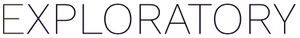# How to apply a function to each row (iterate)?

#1

We are working on a Monte Carlo simulation and have gotten to the point where we have a script that runs the simulation for one row of the following “Draft_DMR” data frame (top three have different permit numbers):

Here is the code for the R script data frame:

``````row=1
runs <-10000
permit <- Draft_DMR\$permit[row]
bod_log_mean <-Draft_DMR\$bod_log_mean[row]
bod_log_sd <- Draft_DMR\$bod_log_sd[row]
nh3_log_mean <- Draft_DMR\$nh3_log_mean[row]
nh3_log_sd <- Draft_DMR\$nh3_log_sd[row]
flow_log_mean <- Draft_DMR\$flow_log_mean[row]
flow_log_sd <- Draft_DMR\$flow_log_sd[row]

sims <- data.frame("permit" = permit,"bod" = qlnorm((runif(runs,0,1)), mean=bod_log_mean, sd=bod_log_sd), "nh3" = qlnorm((runif(runs,0,1)), mean=nh3_log_mean, sd=nh3_log_sd),"flow" = qlnorm((runif(runs,0,1)), mean=flow_log_mean, sd=flow_log_sd))

sims
``````

This script outputs a nice data frame for the specified row (all have the same permit number).

The thing is we have 500+ permits (rows) to run this script for, and I would appreciate some direction relative to iterating this process? In my limited research, it seems that something from the apply() family of functions might come in handy here?

Thanks in advance!

#2

You can use the Summarize step. You can set the ‘permit’ to Group By and add a custom function for bod, nh3 and flow. Each function can have the following expression. (Here I set 10 for iteration for test.)

``````qlnorm((runif(10,0,1)), mean=bod_log_mean, sd=bod_log_sd)
``````

2 Likes
#3

Kei this is exactly what I needed. Thank you so much. It works beautifully.

1 Like